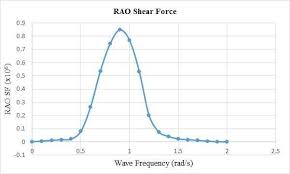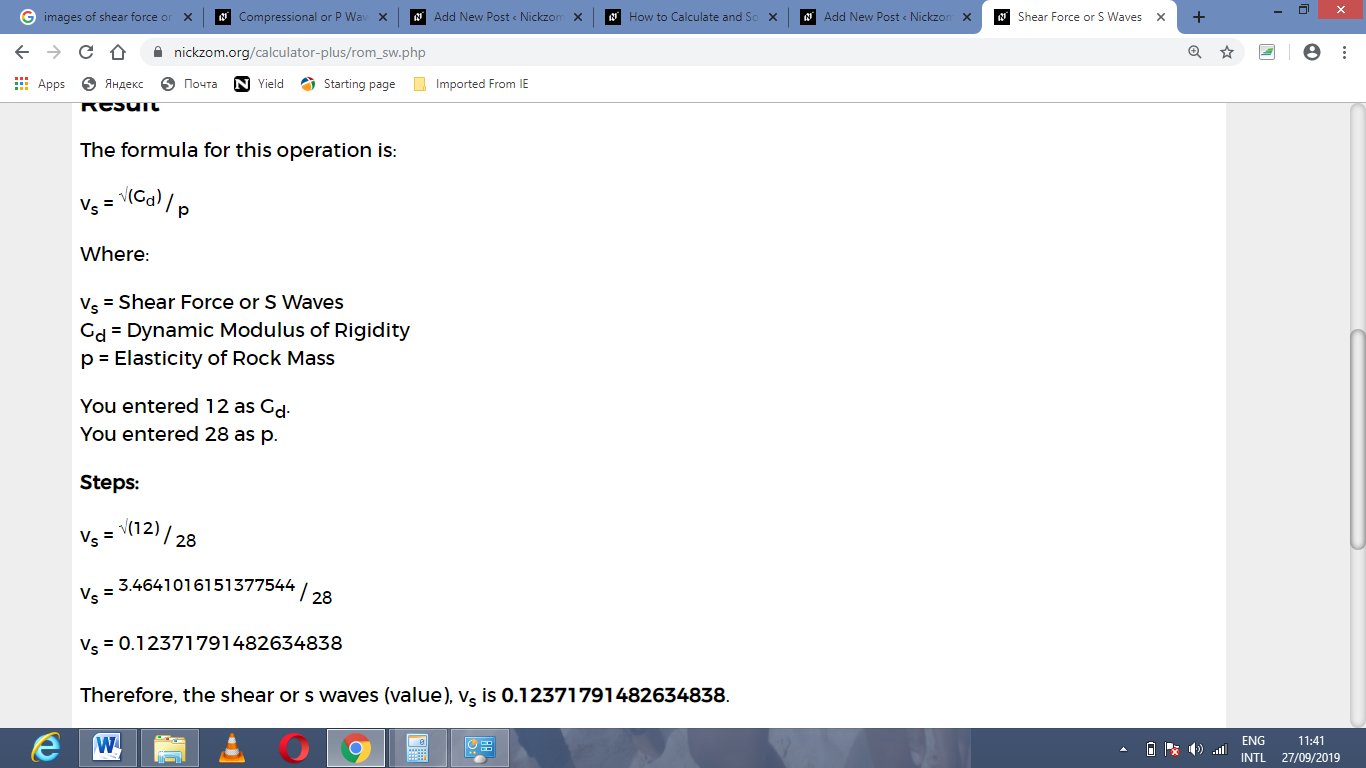How to Calculate and Solve for Shear Force or S Waves | Rock MechanicsThe image above represents shear force or S waves.

To compute for shear force or S waves, two essential parameters are needed and these parameters are Dynamic Modulus of Rigidity (Gd) and Elasticity of Rock Mass (p).

The formula for calculating shear force or S waves:

vs = √(Gd) / p

Where:

vs = Shear Force or S Waves
Gd = Dynamic Modulus of Rigidity
p = Elasticity of Rock Mass

Let’s solve an example;
Find the shear force or s waves when the dynamic modulus of rigidity is 12 and the elasticity of rock mass is 28.

This implies that;

Gd = Dynamic Modulus of Rigidity = 12
p = Elasticity of Rock Mass = 28

vs = √(Gd) / p
vs = √(12) / 28
vs = 3.46 / 28
vs = 0.12

Therefore, the shear force or S waves is 0.12.

Calculating the Dynamic Modulus of Rigidity when the Shear Force or S Waves and the Elasticity of Rock Mass is Given.

Gd = (vs x p)2

Where:

Gd = Dynamic Modulus of Rigidity
vs = Shear Force or S Waves
p = Elasticity of Rock Mass

Let’s solve an example;
Find the dynamic modulus of rigidity when the shear force or s waves is 21 and the elasticity of rock mass is 14.

This implies that;

vs = Shear Force or S Waves = 21
p = Elasticity of Rock Mass = 14

Gd = (vs x p)2
Gd = (21 x 14)2
Gd = (294)2
Gd = 86436

Therefore, the dynamic modulus of rigidity is 86436.

Calculating the Elasticity of Rock Mass when the Shear Force or S Waves and the Dynamic Modulus of Rigidity is Given.

p = √(Gd) / vs

Where:

p = Elasticity of Rock Mass
vs = Shear Force or S Waves
Gd = Dynamic Modulus of Rigidity

Let’s solve an example;
Find the elasticity of rock mass when the shear force or s waves is 24 and the dynamic modulus of rigidity is 10.

This implies that;

vs = Shear Force or S Waves = 24
Gd = Dynamic Modulus of Rigidity = 10

p = √(Gd) / vs
p = √(10) / 24
p = 3.16 / 24
p = 0.13

Therefore, the elasticity of rock mass is 0.13.

Nickzom Calculator – The Calculator Encyclopedia is capable of calculating the shear force or S waves.

To get the answer and workings of the shear force or S waves using the Nickzom Calculator – The Calculator Encyclopedia. First, you need to obtain the app.

You can get this app via any of these means:

To get access to the professional version via web, you need to register and subscribe for NGN 1,500 per annum to have utter access to all functionalities.
You can also try the demo version via https://www.nickzom.org/calculator

Android (Paid) – https://play.google.com/store/apps/details?id=org.nickzom.nickzomcalculator
Android (Free) – https://play.google.com/store/apps/details?id=com.nickzom.nickzomcalculator
Apple (Paid) – https://itunes.apple.com/us/app/nickzom-calculator/id1331162702?mt=8
Once, you have obtained the calculator encyclopedia app, proceed to the Calculator Map, then click on Geology under Add-on.Now, Click on Rock Mechanics under GeologyNow, Click on Shear Force or S Waves under Rock MechanicsThe screenshot below displays the page or activity to enter your values, to get the answer for the shear force or S waves according to the respective parameters which are the Dynamic Modulus of Rigidity (Gd) and Elasticity of Rock Mass (p).Now, enter the values appropriately and accordingly for the parameters as required by the Dynamic Modulus of Rigidity (Gd) is 12 and Elasticity of Rock Mass (p) is 28.Finally, Click on CalculateAs you can see from the screenshot above, Nickzom Calculator– The Calculator Encyclopedia solves for the shear force or S waves and presents the formula, workings and steps too.